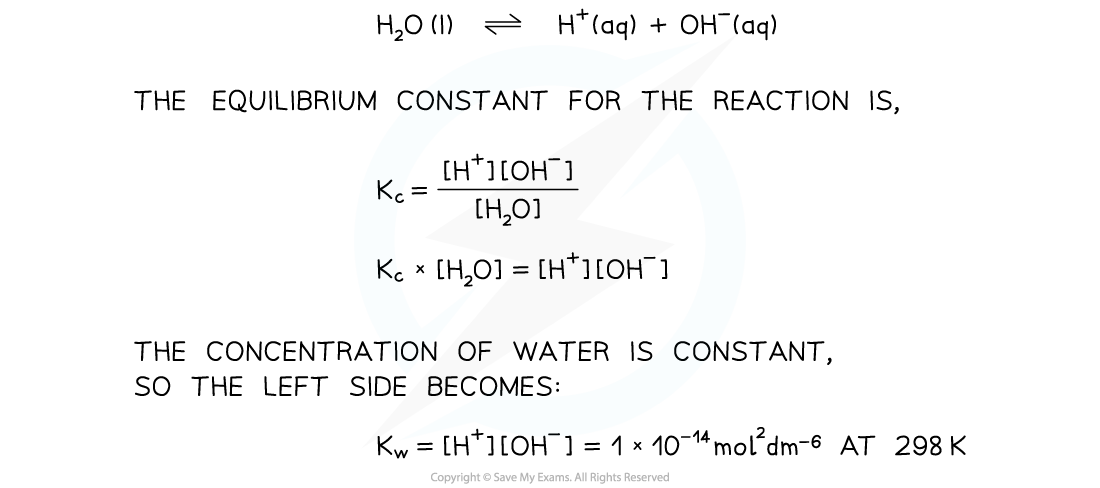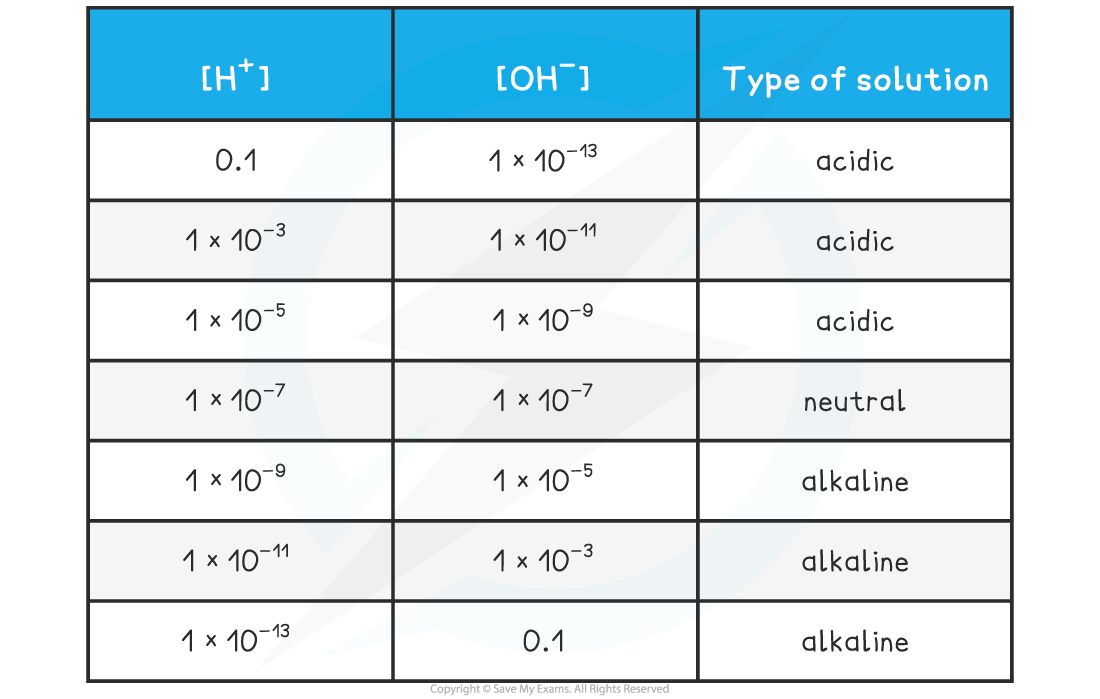# Edexcel A Level Chemistry:复习笔记5.2.4 Ionic Product of Water

### Ionic Product of Water, Kw

• In all aqueous solutions, an equilibrium exists in water where a few water molecules dissociate into protons and hydroxide ions
• We can derive an equilibrium constant for the reaction:• This is a specific equilibrium constant called the ionic product for water
• The product of the two ion concentrations is always 1 x 10-14 mol2 dm-6
• This makes it straightforward to see the relationship between the two concentrations and the nature of the solution:

[H+] & [OH–] TableThe relationship between Kw and pKw is given by the following equation:

pKw = -logKw

#### pKa

• The range of values of Ka is very large and for weak acids, the values themselves are very small numbers

Table of Ka values• For this reason it is easier to work with another term called pKa
• The pKa  is the negative log of the Ka value, so the concept is analogous to converting [H+] into pH values

pKa = -logKa

• Looking at the pKa values for the same acids:

Table of pKa values• The range of pKa values for most weak acids lies between 3 and 7

### pH Calculation of a Strong Base

• Strong bases are completely ionised in solution

BOH (aq) → B+ (aq) + OH- (aq)

• Therefore, the concentration of hydroxide ions [OH-] is equal to the concentration of base [BOH]
• Even strong alkalis have small amounts of H+ in solution which is due to the ionisation of water
• The concentration of OH- in solution can be used to calculate the pH using the ionic product of water
• Once the [H+] has been determined, the pH of the strong alkali can be founding using pH = -log[H+]• Similarly, the ionic product of water can be used to find the concentration of OH- ions in solution if [H+] is known, simply by dividing Kw by the [H+]

#### Worked Example

pH calculations of a strong alkali

Question 1:

Calculate the pH of 0.15 mol dm-3 sodium hydroxide, NaOH

Question 2:

Calculate the hydroxide concentration of a solution of sodium hydroxide when the pH is 10.50

Sodium hydroxide is a strong base which ionises as follows:

NaOH (aq) → Na+ (aq) + OH- (aq)

The pH of the solution is:

[H+] = Kw  ÷ [OH-]

[H+] = (1 x 10-14) ÷ 0.15 = 6.66 x 10-14

pH = -log[H+]

= -log 6.66 x 10-14  = 13.17

Step 1: Calculate hydrogen concentration by rearranging the equation for pH

pH = -log[H+]

[H+]= 10-pH

[H+]= 10-10.50

[H+]= 3.16 x 10-11 mol dm-3

Step 2: Rearrange the ionic product of water  to find the concentration of hydroxide ions

Kw = [H+] [OH-]

[OH-]= Kw  ÷  [H+]

Step 3: Substitute the values into the expression to find the concentration of hydroxide ions

Since Kw is 1 x 10-14 mol2 dm-6,

[OH-]= (1 x 10-14)  ÷  (3.16 x 10-11)

[OH-]= 3.16 x 10-4 mol dm-3